HELLO, GUEST## Computable Numbers: The Turing Machine

Louise Scupham

This essay will explore the Turing machine and its relationship with computable numbers and an introduction to real numbers of various types. I will offer an explanation of the reasons why a number may or may not be considered computable through the use of the concept of countable sets and what makes a set countable.

Computable numbers consist of any real number which can be computed to desired precision by a finite terminating algorithm (Anon., 2016).

It must be noted that this does not mean that all real numbers are computable, just that to be computable a number must be real. Which numbers are in fact computable is something that will be discussed later in this essay.

To fully understand this definition I will first define some of the key terms mentioned within it.

A real number is any number that could be expressed on a conventional number line. This includes the natural numbers, integers, rational numbers and irrational numbers.

Natural numbers are positive integers such as 1, 2, 3, 4, 5 etc. Integers consist of all whole numbers, so the natural numbers and their negative counterparts e.g. -3, -2, -1, 0, 1, 2, 3 (MathPlanet, n.d.). Lying between the integers on the number line we have non-integer numbers, which can be either rational or irrational.

Rational numbers can be expressed as a fraction of integer numbers such as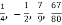etcetera. All natural and integer numbers are considered rational, for example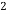can be expressed as a fraction of integersso is both a rational and an integer.

Irrational numbers cannot be expressed as a fraction of integers and include numbers such as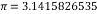â€¦ and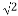.

In essence then, almost any number we can think of is a real number.

The standard definition of an algorithm is a process or set of rules followed during a calculation (Oxford English Dictionary, 2017). However, a definition more specific to computing describes an algorithm as a procedure that allows a computer to solve a problem. They consist of a set of unambiguous instructions, meaning that each time a computer carries out the same algorithm it will produce exactly the same result (Zandbergen, 2003).

If you need assistance with writing your essay, our professional essay writing service is here to help!

So a computable number is, in essence, one which can be written by a finite computer program, where the finality refers to the set of instructions rather than the number of steps carried out (Hadley, n.d.).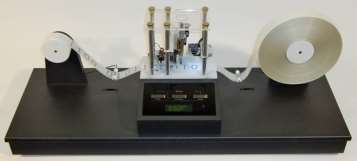A Turing machine is an idealised, hypothetical computing device conceived by the mathematician Alan Turing. It has the capability of simulating any computer algorithm, no matter how complicated (Mullins, 2012) and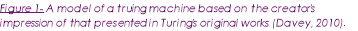consists of a read/write head as well as paper tape. This tape is of infinite length and divided into squares which may contain symbols or remain blank. The purpose of this tape is to serve as a general storage medium for both input and output and acts as a working memory to store intermediate steps of the computation (Copeland, 2000).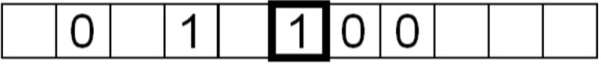In the simple example given in figure 2 (below) the only symbols that the machine can process are 1, 0, or blank and because of this it is referred to as a three symbol Turing machine (Mullins, 2012).

Figure 2 A simple representation of a length of the infinite tape of a 3 symbol Turing machine (Mullins, 2012)

The head is placed over a square on the tape and carries out operations as instructed by the computer program. The square selected by the head in figure 1 is the number 1, made identifiable by the emboldened square surrounding it. The head contains a sub-device, which will be referred to as the indicator, the indicator can be set to any one of a number of positions, and its position at any given time is referred to as the state of the Turing machine.

So having identified the key components of the Turing machine we must now understand how it works.

There are a few main types of fundamental operation which the machine is able to perform. These are the ‘instructions’ which were mentioned previously and are more formally known as primitive or atomic operations (Copeland, 2000). These ‘atoms’ are listed below.

2. Erase the symbol underneath the head and replace it with another (Mullins, 2012)
3. Move the tape left or right by one square
4. Change State
5. Halt (Copeland, 2000)

Based solely on these operations a simple computer program can be constructed, for example inversion could be performed on the tape displayed in figure 3 (Mullins, 2012), where the 1’s are changed to 0’s and visa versa.

This is done by giving the following instruction to the Turing machine as seen in table 1.

 Symbol Write Move Blank None None 0 Write 1 Move tape to the right 1 Write 0 Move tape to the right

Table 1 shows the instructions that must be followed by the Turing machine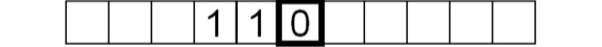The machine will read the symbol under the head first, which in this case is 0 (highlighted by a box in figure 3 below). It will then perform the task, move the tape and repeat as instructed (Mullins, 2012).

Figure 3– Another section of tape from a 3 symbol Turing Machine, the emboldened box represents that which the machine head rests above (Mullins, 2012).

The machine will first rewrite 0 as 1, then move one square to the right to sit above the middle square containing the ‘1’ symbol. It will then change this 1 to 0, move one square to the right and perform the same operation on final ‘1’ square. This will result in figure 3 (1, 1, 0) being altered and becoming figure 3.1 (not shown) reading 0, 0, 1. This program will effectively end upon reaching the blank square, since the instruction for encountering a blank symbol states that no further operation is to be performed.

Modern computers have a much greater number of primitive operations or atoms which are vastly more complicated, but, despite its incredible simplicity, the Turing machine is able to compute more than any physical computer (Copeland, 2000).

In many instances programs run by a Turing machine will be terminating, meaning that it will reach a halt atom no matter what the input. Such an example was the one which I described above in the inversion of symbols on the tape seen in figure 3. However this is not always the case. An example of a non-terminating Turing machine is the one which programmed to calculate pi (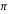) digit by digit, this program will run for eternity and never reach a halt point (Copeland, 2000).

Any number that can be written out by a Turing machine is computable, which explains why some irrational numbers such as pi are computable.

One would assume that all real numbers are also computable numbers. At first it would appear to be a quite logical assumption that a Turing machine could write out any number with a decimal representation (any real number) (Copeland, 2000). This, however, is not the case and in fact quite the opposite is true.

There are relatively few computable numbers among the real numbers (Copeland, 2000) and to understand why this is another concept must be introduced and this is the idea of countable sets.

A countable set, is set of numbers, which may be finite or infinite, but can always be counted one at a time, even though counting may never finish in the case of an infinite set (Karagila, 2016). Every element in this countable set is associated with a natural number, known as one-to-one correspondence (Karagila, 2016) (Hadley, n.d.).

To further explain one-to-one correspondence I will put this into context with an example.

If we had a number of cats (C) and a number of mice (M) and we wanted to compare the sizes of these two sets, a logical method would be to assign one mouse to each cat. If we any cats did not have a mouse to eat we would know that C>M. If any mice were running free we would know that M>C and if each cat had one mouse and only one mouse, then M=C.

If we were to think of the Cats in this analogy as the natural numbers and the mice as the elements to be counted, then matching up the mice with a cat is putting the elements of two sets into one-to one correspondence.

If however there are more mice than cats, that is more elements than countable numbers, then that set is not countable, as it cannot be put into one-to-one correspondence.

From this we can clearly draw the conclusion that the natural numbers are countable. But what about the integers?

If the integers consist of the natural numbers and their negative counterparts, it could be easily assumed that there are more integers than the natural numbers (nearly twice the amount in fact) which would render them uncountable (Körner, 2011). For a start, if we were to count the integers in order of magnitude starting with the least, there would be an infinite amount of negative numbers so we would never reach 0 to then count the positive numbers (Körner, 2011).

If however we order the integers in a differently, as shown in table 2, we find that they are in fact countable.

 N= 1 2 3 4 5 6â€¦ integer 0 -1 1 -2 2 -3â€¦

Table 2 – how integers may be ordered to achieve one-to-one correspondence

Imagine counting the integers and reaching a point on the list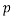. Any integer with an absolute value smaller than that of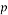will have been counted exactly once (Körner, 2011).

Take integer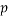to be -3 for example. The absolute value of -3 (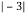) = 3 and as can be seen from table 2 all integers with an absolute value less than 3 have already been counted and exactly once.

Yet if there appears to be two integers (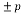) for each natural number how can one-to-one correspondence be achieved?

This assumption is based on the belief that infinity is a number, when it quite simply is not. Take the example of a length of string an infinite number of cm’s long. Considering infinity as a number in this situation creates a paradox. An infinitely long line would have a length of infinity centimetres, and so would also have a length of infinity miles, yet since a centimetre is a much smaller than a mile the line must be two different lengths simultaneously (Körner, 2011). An impossible notion.

Our academic experts are ready and waiting to assist with any writing project you may have. From simple essay plans, through to full dissertations, you can guarantee we have a service perfectly matched to your needs.

But in reality, the centimetres that are counted to make the infinitely many miles can be put into one-to-one correspondence with the centimetres that are counted to reach infinitely many centimetres (Körner, 2011), a concept which when applied to integers shows that they are countable.

Then we are lead to rational numbers. All rational numbers are countable, but again at first it would appear to be the opposite.

There are an infinite number of rational numbers, just as there is an infinite number of natural number, integers and real numbers (Wladis, n.d.) and between any two rational numbers there is always another (Körner, 2011).

As we have already established all real numbers can be expressed on the number line. If we were to take any two of the integers on this line (which are also rational numbers) say 2 and 3, we would find there are rational numbers between them for example 2.1, 2.2, 2.3 and so on. But we could break that down further 2.01, 2.02, 2.03 and again 2.001 and again 2.0001 and again 2.0000001â€¦ and we could break it down using integer factors other 10 such as 2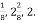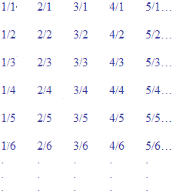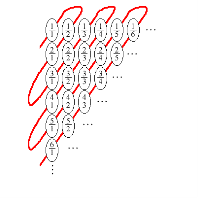To get all the rational numbers you would have to take each integer one at a time and divide it in turn by each natural number, which is shown in figure to the right. Since there are infinitely many natural numbers, and infinitely many integers it would appear that there should be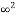number of rational numbers. But this of course is not possible, because this is based on the assumption that infinity is a number, which we know not to be true (Körner, 2011).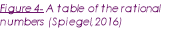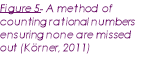Ordering rationals is not an easy task as can be seen in figure and some rationals are counted more than once, for example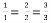, but as long as they can be lined up in an order then they can be put into one-to-one correspondence with the natural number so are therefore countable (Spiegel, 2016).

So finally we will consider irrational numbers and their countability.

We can prove that irrational numbers are not countable by contradiction. So far we have shown that all real numbers (with the exception of the irrationals) are countable so we can assume that if the real numbers are countable then the irrational numbers must be also. Equally if the real numbers are proved to be uncountable then the irrationals must be the cause of this.

So supposing the real numbers are countable we would try to put them into on-to-one correspondence, and example of part of this list is below in table 3.

 1 2 3 4 5â€¦ 0.123568 1.14368 2.39851 3.37852 4.145669

Table 3– part of the list of real numbers in one-to-one correspondence

Theoretically every real number should occur somewhere in this infinite list. However if we were to take the first digit after the decimal point from the first number, the second digit from the second number etc. etc. you would get a new numberâ€¦ 0.14856. by adding 1 to each digit in this new number (0.25967) we will get a number, which is not the same as any number on the listâ€¦ it is not the same as the first because their first decimal digits are different, nor the second, because their second decimal digits are different and so on. This means that this number is not already on the list, a list which we had assumed contained every real number. Clearly then the number of real numbers is greater than that of the natural numbers, meaning one-to-one correspondence cannot be achieved and the real number are therefore not countable. In this way we also learn that not all infinities are the same size, a confusing notion, but this example clearly proves that an uncountable infinity is larger than one which is countable (mf344, 2013).

Since the real numbers are not countable, the irrational numbers are also uncountable, but what does this mean in terms of the computability of numbers and Turing machines?

Knowing that real numbers are not countable shows that that not all real numbers are computable and this is because there are a countable number of Turing machines.

Based on this we can see that there will always be more real numbers than the number of Turing machines. Each Turing machine can write one number, so if there are more real numbers than numbers than Turing machines, and Turing machines can write all the computable numbers, then there must be more real numbers than computable numbers. So not all real numbers can be computable.

There are a countable number of rationals, integers and natural numbers and as a result all of these numbers are computable, however the irrationals are not countable, but this does not mean that they are all incomputable.

Turing was able to prove that some irrational numbers are simply not computable because their digits are so lacking in a pattern that they cannot be written using a finite table of instructions as required by a Turing machine (Copeland, 2000).

Yet some irrationals are computable such as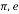and the square root of integers, among others which can be defined in a reasonable manner (Su, 1999)

Conclusions

Turing machines are an incredible concept which is able to simulate any algorithm or produce any computable number.

Not all real numbers are computable, in fact in the grand scheme of things very few are. Through the concept of countable sets it can be shown that all integers, natural numbers and rational numbers are countable, along with some irrational numbers such asand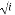whereis an integer. However many irrational numbers are not computable because they are lacking in pattern and therefore cannot be written using a finite terminating algorithm. Therefore the real numbers are not a countable set and since there are a countable number of Turing machines, each capable of producing a computable number, then all real numbers cannot be computable. Any set of numbers that is countable is also computable.

References

Anon., 2016. Commputable number. [Online]
Available at: https://en.wikipedia.org/wiki/Computable_number
[Accessed 5 March 2017].

Copeland, J., 2000. What is a Turing Machine?. [Online]
Available at: http://www.alanturing.net/turing_archive/pages/reference%20articles/what%20is%20a%20turing%20machine.html
[Accessed March 13 2017].

Davey, M., 2010. A Turing Machine. [Online]
Available at: http://aturingmachine.com/
[Accessed 2017 March 13].

Hadley, P., n.d. Uncomputable numbers. [Online]
[Accessed 2017 March 13].

Karagila, A., 2016. Why isn’t the set of real numbers countable. [Online]
Available at: http://math.stackexchange.com/questions/1673582/why-isnt-the-set-of-real-numbers-countable
[Accessed 21 March 2017].

Körner, K., 2011. All about infinity. [Online]
Available at: https://nrich.maths.org/2756
[Accessed 21 March 2017].

MathPlanet, n.d. Exploring real numbers/integers and rational numbers. [Online]
Available at: http://www.mathplanet.com/education/algebra-1/exploring-real-numbers/integers-and-rational-numbers
[Accessed 21 March 2017].

mf344, 2013. Countable Infinities. [Online]
Available at: https://plus.maths.org/content/maths-minute-countable-infinities
[Accessed 21 March 2017].

Mullins, R., 2012. Turing Machine. [Online]
Available at: https://www.cl.cam.ac.uk/projects/raspberrypi/tutorials/turing-machine/one.html
[Accessed 8 March 2017].

Oxford English Dictionary, 2017. Algortihm. [Online]
Available at: https://en.oxforddictionaries.com/definition/algorithm
[Accessed 5 March 2017].

Spiegel, J., 2016. Non Computable Numbers. [Online]
Available at: http://www.i-programmer.info/babbages-bag/1902-non-computable-numbers.html
[Accessed 5 March 2017].

Su, F., 1999. Computability of Real Numbers. [Online]
Available at: https://www.math.hmc.edu/funfacts/ffiles/30004.8.shtml
[Accessed 22 March 2017].

Wladis, C., n.d. Inifinite sets. [Online]
[Accessed 21 March 2017].

Zandbergen, P., 2003. What is a Computer Algorithm? – Design, Examples & Optimization. [Online]
[Accessed 5 March 2017].## Most Used Categories

Recommendation
With Our Resume Writing Help, You Will Land Your Dream Job
Resume Writing Service, Resume101
Trust your assignments to an essay writing service with the fastest delivery time and fully original content.
Essay Writing Service, EssayPro
Nowadays, the PaperHelp website is a place where you can easily find fast and effective solutions to virtually all academic needs
Universal Writing Solution, PaperHelp
Professional Custom
Professional Custom Essay Writing Services
In need of qualified essay help online or professional assistance with your research paper?
Browsing the web for a reliable custom writing service to give you a hand with college assignment?
Out of time and require quick and moreover effective support with your term paper or dissertation?